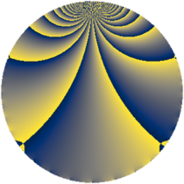# Properties

 Label 1148.2.cLevel $1148$ Weight $2$ Character orbit 1148.c Rep. character $\chi_{1148}(1147,\cdot)$ Character field $\Q$ Dimension $164$ Sturm bound $336$

# Learn more about

## Defining parameters

 Level: $$N$$ $$=$$ $$1148 = 2^{2} \cdot 7 \cdot 41$$ Weight: $$k$$ $$=$$ $$2$$ Character orbit: $$[\chi]$$ $$=$$ 1148.c (of order $$2$$ and degree $$1$$) Character conductor: $$\operatorname{cond}(\chi)$$ $$=$$ $$1148$$ Character field: $$\Q$$ Sturm bound: $$336$$

## Dimensions

The following table gives the dimensions of various subspaces of $$M_{2}(1148, [\chi])$$.

Total New Old
Modular forms 172 172 0
Cusp forms 164 164 0
Eisenstein series 8 8 0

## Trace form

 $$164q - 4q^{2} - 4q^{4} - 16q^{8} - 164q^{9} + O(q^{10})$$ $$164q - 4q^{2} - 4q^{4} - 16q^{8} - 164q^{9} - 4q^{16} - 156q^{25} - 44q^{32} - 68q^{36} - 8q^{37} + 40q^{42} + 64q^{46} - 12q^{49} - 24q^{50} + 16q^{57} - 40q^{64} + 2q^{72} + 74q^{74} - 32q^{77} + 42q^{78} + 228q^{81} - 10q^{84} - 4q^{86} + 74q^{92} + 4q^{98} + O(q^{100})$$

## Decomposition of $$S_{2}^{\mathrm{new}}(1148, [\chi])$$ into newform subspaces

The newforms in this space have not yet been added to the LMFDB.